# Part 4: The Cartesian Plane

Are you confused about coordinate planes? Well, don't worry! We will show you how to plot points on a Cartesian plane, find coordinates of a specific point and draw linear relationships on a Cartesian plane.## What is the Cartesian Plane?

The Cartesian Plane provides a method of representing pairs of numbers that are related, as coordinate points.

If we plot multiple points, we can represent general mathematical relationships such as linear equations.

## NSW Syllabus Outcomes

 Syllabus Explanation NSW Stage 4 NESA Syllabus Given coordinates, plot points on the Cartesian plane, and find coordinates for a given point (ACMNA178) Plot and label points on the Cartesian plane, given coordinates, including those with coordinates that are not whole numbers.Identify and record the coordinates of given points on the Cartesian plane, including those with coordinates that are not whole numbers Plot linear relationships on the Cartesian plane, with and without the use of digital technologies (ACMNA193) Form a table of values for a linear relationship by substituting a set of appropriate values for either of the pronumerals and graph the number pairs on the Cartesian planeExtend the line joining a set of points on the Cartesian plane to show that there is an infinite number of ordered pairs that satisfy a given linear relationshipRead coordinates from the graph of a linear relationshipDerive a rule for a set of points that has been graphed on the Cartesian plane

## Assumed knowledge

Students should be confident with basic algebraic techniques such as substitution and have a basic understanding of linear relationships.

## Using the Cartesian Plane to find coordinates

The cartesian plane is a grid which can be used to represent points.

Each point is a pair of numbers with an $$x$$ and $$y number. Eg. A $(2,5)$$ means for a point $$A$$, the $$x$$ coordinate is $$2$$ and the $$y$$ coordinate is $$5$$ :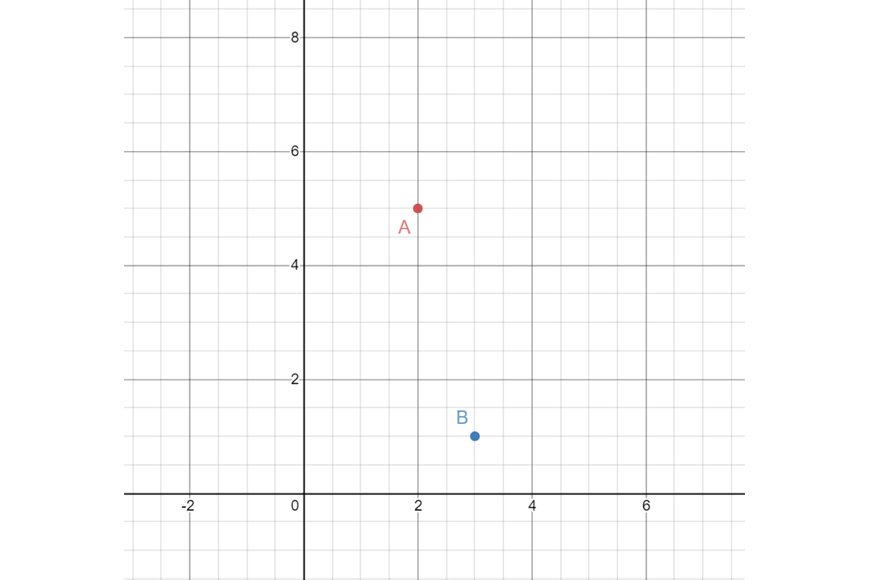Conversely, we can find the coordinates of a point B$ on the plane:

• $$x$$ coordinate is $$3$$
• $$y$$ coordinate is $$1$$

So, we write $$B(1,3)$$.

When we are given a linear relationship such as $$y = 5 + x$$, we can represent this on a cartesian plane by determining which points the relation goes through and joining them to form a straight line.

To do this, first we create a table of values:

 x 0 1 2 3 4 y 5 6 7 8 9

Then we can plot each point on the coordinate plane, and draw a line that goes through these points: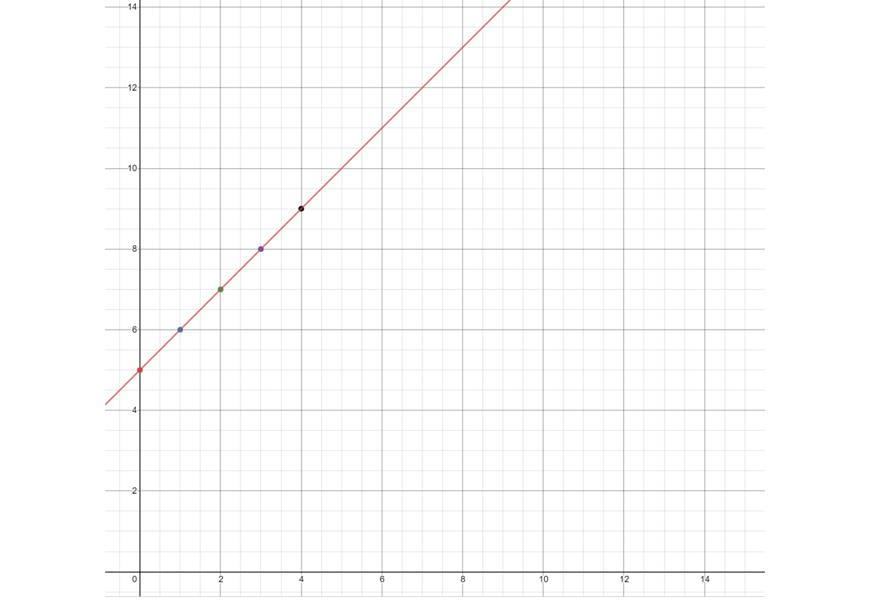Any points that lie on the line (even those that aren’t points on the grid) are solutions to the linear relationship $$y=5+x$$.

The line continues forever, so there are infinite solutions to this relation.

For example, we can see that the point $$(5,10)$$ lies on the line, and so when $$x=5$$, $$y=10$$, we have $$10 = 5 + 5$$.

We can also determine linear relationships based on lines on a cartesian plane.

For example, consider the following line: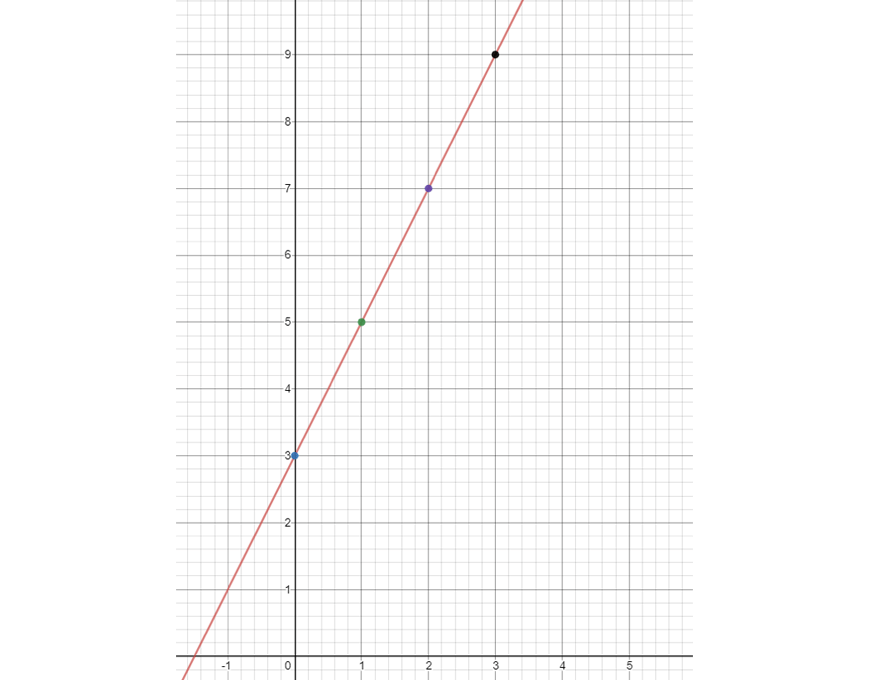Looking at the points marked on the line, we can construct the following table:

 $$x$$ 0 1 2 3 $$y$$ 3 5 7 9

What is the equation represented by this line?

We know that when $$x = 0$$, $$y = 3$$; and the difference between successive $$y$$ values is  $$2$$.

So, therefore our equation must be  $$y = 3 + 2x$$.

## Summary

Now we have covered reading points from a graph, constructing a line on the cartesian plane by plotting values, and finding the equation that is represented by a given line on the coordinate axes.

## Want to see how well you know the Coordinate Plane?## Checkpoint Questions

1. Plot the following points on the cartesian plane:

a. (1,2)

b. (2,3)

c. (-1,4)

2. Given $$y = 3x-x$$, find 3 points on the line and hence plot the line.

3. Determine the linear relation governing the following line.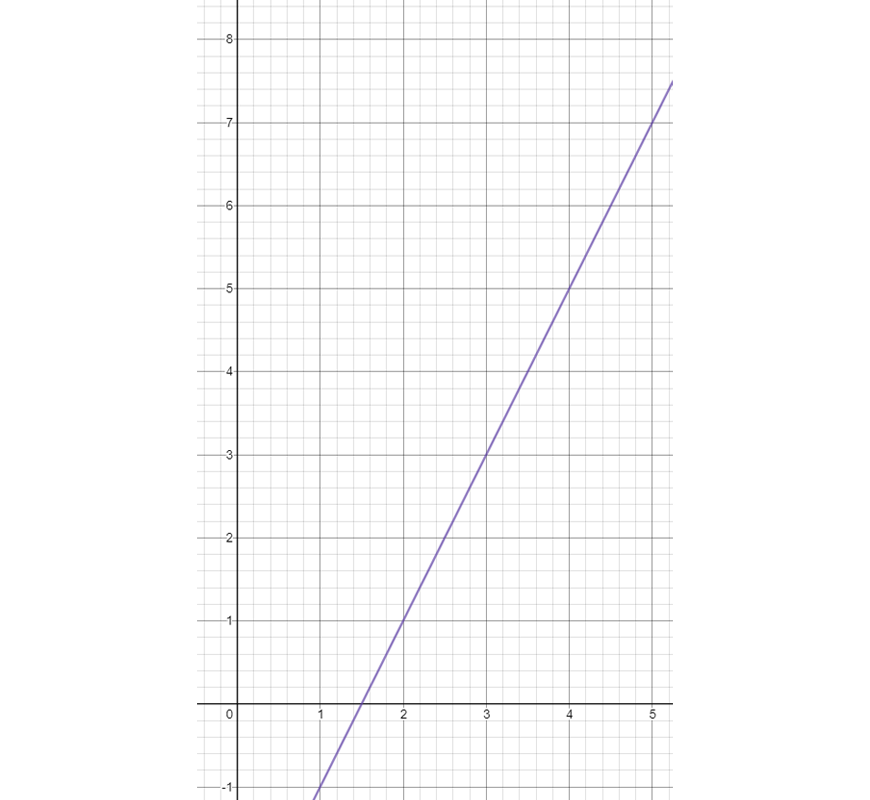## Solutions:

1)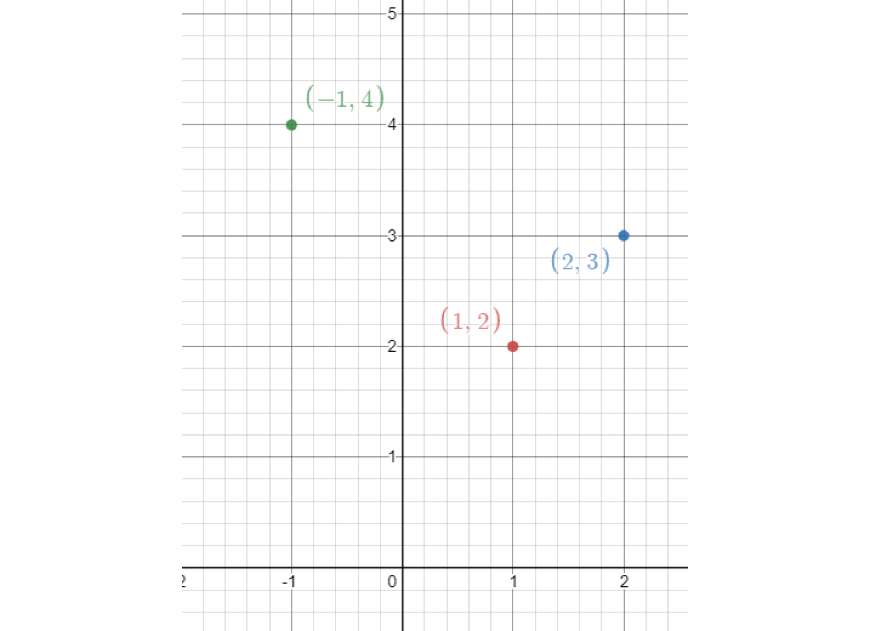2)

 $$x$$ 0 1 2 $$y$$ 3 6 9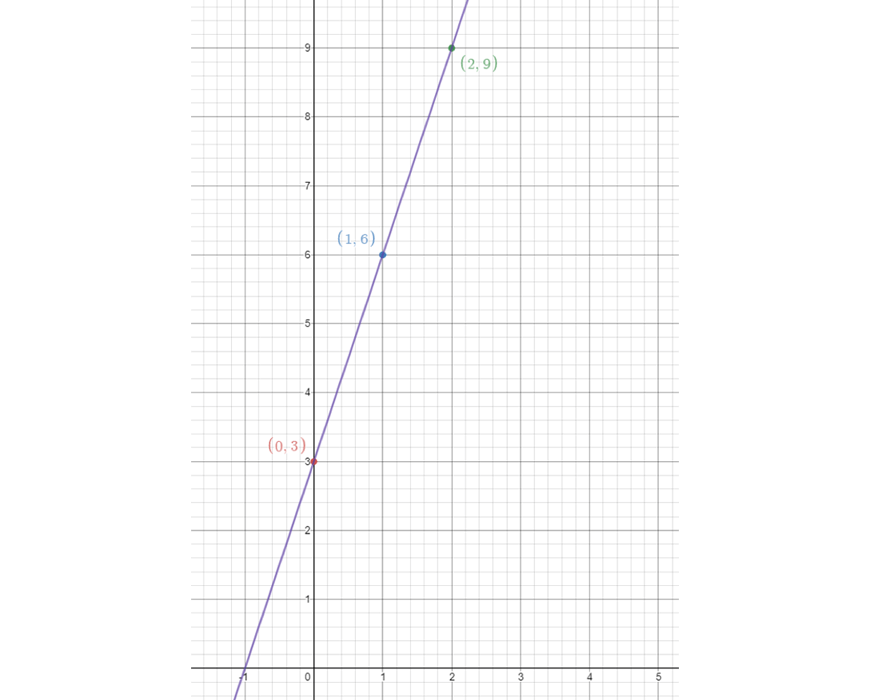3) $$y = 2x – 3$$

© Matrix Education and www.matrix.edu.au, 2021. Unauthorised use and/or duplication of this material without express and written permission from this site’s author and/or owner is strictly prohibited. Excerpts and links may be used, provided that full and clear credit is given to Matrix Education and www.matrix.edu.au with appropriate and specific direction to the original content.